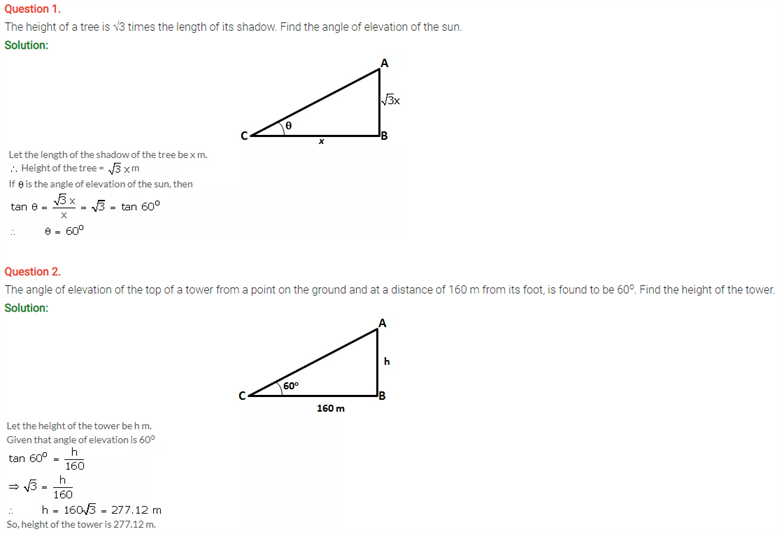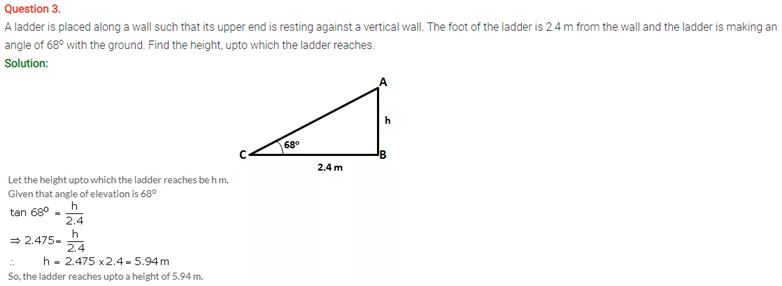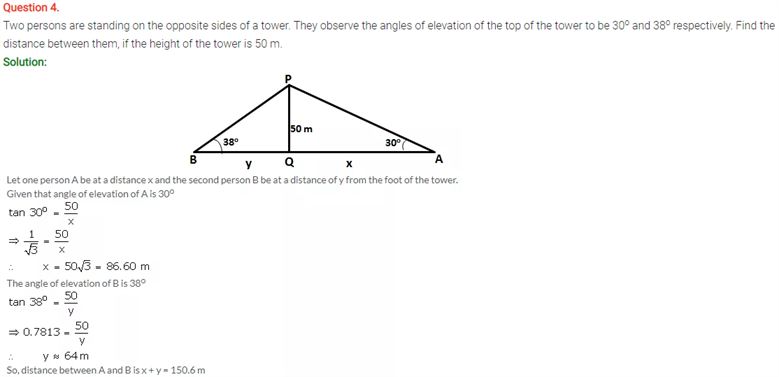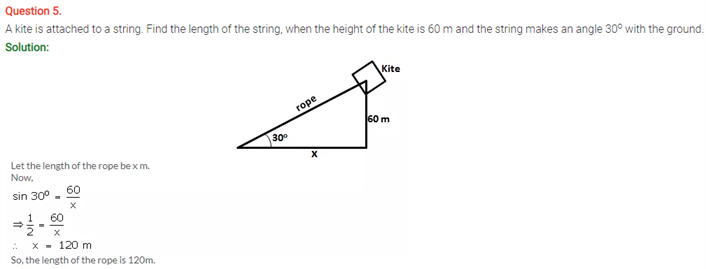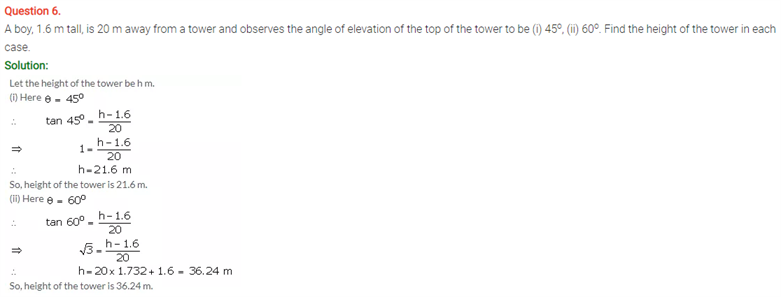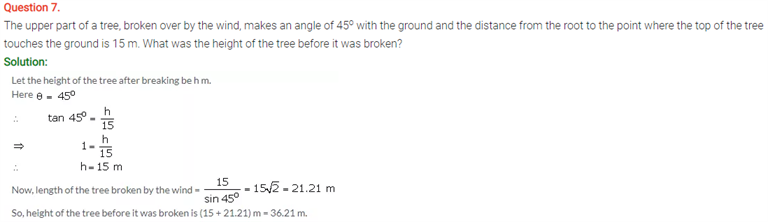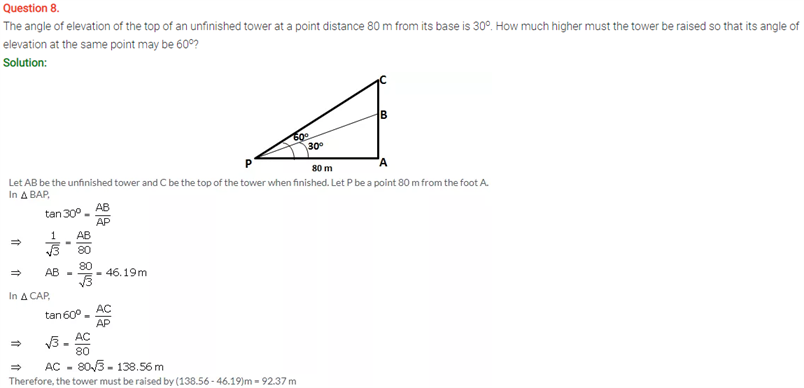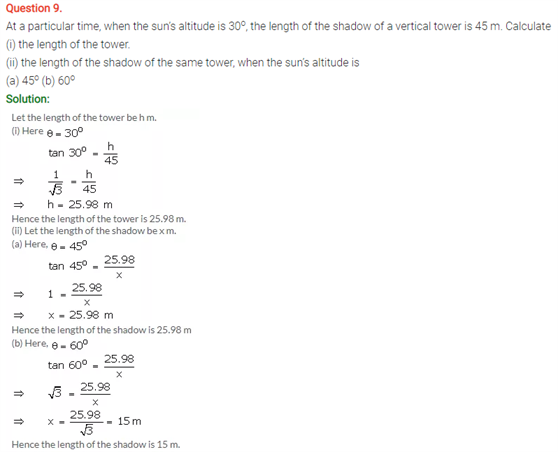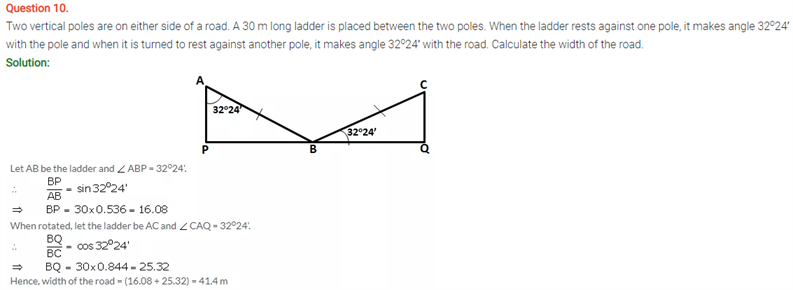# Selina Concise Maths Solution for ICSE Class 10 Chapter 22 Heights and Distances

## Selina Class 10 Maths Solutions for Chapter 22

ICSE Solutions For Class 10 Maths brings you the questions on the application of trigonometry. This chapter consists of a variety of topics in which problems are expected in exams. The two major concepts are the angle of elevation which is if the object is above the horizontal level from the eye we have to lift up our head to view the object. In this process, our eyes move through the angle.This angle is called the angle of elevation. Another concept is the angle of depression, if the object is below the horizontal level from the eye, we have to move downwards our head to see the object. In this process, our eyes move through an angle.This angle is called the angle of depression. Students will find solutions on these topics.

Check out ICSE Class 10 Maths Selina Solutions Chapter 22 Heights and Distances below: